# How to Calculate Average Ignore Non-Numeric Values and Errors

In daily work we often need to calculate the average of some numbers in a range for further analysis. Thus, we need to know some basic functions of calculate average in Excel. From now on, we will introduce you some basic functions like AVERAGE, AVERAGEIF, and show you some common formulas to calculate average value.

In this article, we will let you know how to calculate average by AVERAGE function easily and the way to calculate average based on a given criteria by AVERAGEIF function. We will introduce you the syntax, arguments, and basic usage about the two functions, and let you know the working process of our created formulas.

## EXAMPLE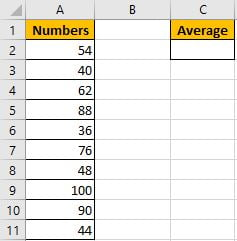Refer to “Numbers” column, some numbers are listed in range A2:A11. C2 is used for entering a formula which can calculate the average of numbers in range A2:A11. In fact, Excel has amount of built-in functions and they can execute most simple calculations properly, and AVERAGE is one of the most common used functions.

In C2, enter “=AVERAGE(A2:A11)”.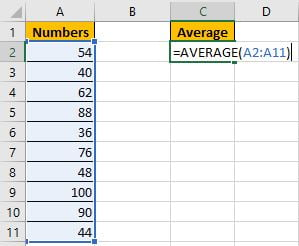Then press Enter, AVERAGE function returns 63.8.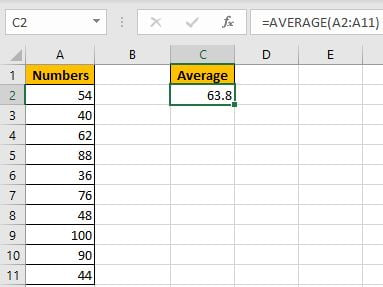You can adjust decimal places by “Increase Decimal” or “Decrease Decimal” in “Number” section.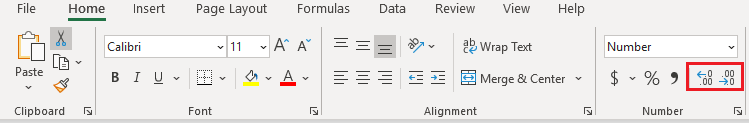But if some errors or blank or non-numeric values exist in the list, how can we only calculate average for numbers ignoring the invalid values?  See example below.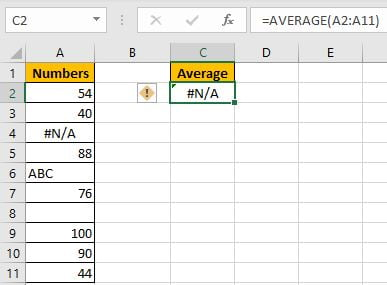We get an error when calculating average for range A2:A11, the difference is there are some invalid values in the list. To calculate average ignoring the errors, that means we need to calculate average of numbers in a range with one or more criteria, thus, we can apply AVERGAEIF function instead of basic AVERAGE function.

## CREATE A FORMULA with AVERAGEIF FUNCTION

### 1. STEPS

Step 1: In C2, enter the formula =AVERAGEIF(A2:A11,”>0″).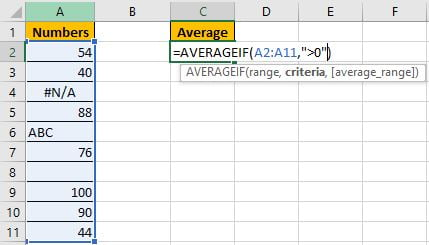You can also name range “Numbers” for A2:A11, then you can enter =AVERAGEIF(Numbers,”>0″).

Step 2: Press Enter after typing the formula.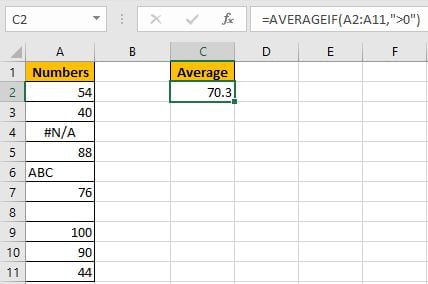Ignore the improper cells, and only calculate the average of numbers 54, 40, 88, 76, 100, 90 and 44, total 7 numbers. (54+40+88+76+100+90+44)/7=70.3. The formula works correctly.

### 2. FUNCTION INTRODUCTION

AVERAGEIF function is AVERAGE+IF. It returns the average of some numbers in a range based on given criteria.

Syntax:

`=AVERAGEIF(range, criteria, [average_range])`

It supports wildcards like asterisk ‘*’ and question mark ‘?’, also supports logical operators like ‘>’,’<’. If wildcards or logical operators are required, they should be enclosed into double quotes (““), in this case we entered “>0” to show the criteria.

### 3. ALL ARGUMENTS

AVERAGEIF – RANGE

This example is very simple, we have only one list. A2:A11 is criteria range and average range.

In the formula bar, select “A2:A11”, press F9, values in this range are expanded in an array.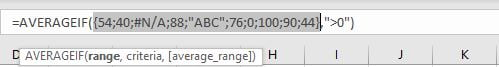If average range=criteria range, average range can be omitted.

AVERAGEIF – CRITERIA

Obviously, the criteria in our case is “>0”, this condition can filter numbers from criteria range.

### 4. HOW THE FORMULA WORKS

After expanding values, the formula is displayed as:

=AVERAGEIF({54;40;#N/A;88;”ABC”;76;0;100;90;44},”>0″)

Note: blank cell is recorded as 0 in the array.

Because of criteria “>0”, so invalid cells are ignored. Replace all invalid values with 0. Keep all numbers.

{54;40;#N/A;88;”ABC”;76;0;100;90;44} -> {54;40;0;88;0;76;0;100;90;44}

Now this new array only contains numbers. We can calculate the average now.

## SUMMARY

1. AVERAGE function is used for returning the average of some numbers in Excel.

2. AVERAGEIF function returns the average of some numbers refer to given criteria.

3. For argument, if range=average range, average range can be omitted.

4. AVERAGEIF can support wildcards, logical operators.

### Related Functions

• Excel AVERAGE function
The Excel AVERAGE function returns the average of the numbers that you provided.The syntax of the AVERAGE function is as below:=AVERAGE (number1,[number2],…)….
• Excel AVERAGEIF function
The Excel AVERAGEAIF function returns the average of all numbers in a range of cells that meet a given criteria.The syntax of the AVERAGEIF function is as below:= AVERAGEIF (range, criteria, [average_range])….

Related Posts

Break ties with helper COUNTIF and column

Suppose you got a task to adjust the values that contain the ties; what would be your first attempt to break the ties of the given value? If you are wondering about doing this task manually, let me add that ...

Calculate Total Cost with Excel VLOOKUP Function

In today's article we will show you how to calculate the total cost for a given weight using the Excel VLOOKUP function. This function will help us to find the appropriate unit price for that weight and then we can ...

Excel Formulas To Calculate The Bond Valuation

Assume that you've been assigned a task of calculating bond valuation; so if you are new to Ms Excel or do not have much experience with it, then I am pretty sure about it that doing this task manually might ...

Calculate Cumulative Totals with Excel SUM Function

Today, through a simple example, we will show you how to use one of the most common-used Mathematical functions in excel, the SUM function, to add up the sum. In our daily life, we keep an account of what we ...

BMI Calculation Formula In Ms Excel

You might have come across a task in which you were assigned to make BMI calculations of the supplied numbers, and you may be looking for an efficient approach to accomplish this process rather than doing BMI calculations manually, by ...

Calculate The Period of Loan or Investment in Excel

In our daily life, most of us will invest or take a loan, so we need to master some simple financial functions to calculate the period needed for loans or investments. If you work as an accountant, you need to ...

Working Time Calculation Based on Timesheets

In the office, a special machine record the time when you start working (clock in office) and when you finish working (clock out of office). We can calculate the total working time by subtracting the end working time from the ...

Sort/Rank Numeric Values with Duplicate Values Exist

Excel built-in RANK function can sort a set of values. If there are duplicate numbers, then the rank number is also duplicated. See the following example: There are two numbers “100” in range A2:A9, they are both the third largest ...

Calculate Days Open in Excel

If you want to know how to Calculate days in Excel, there are some formulas that you can use to do so. For example, you can use the DAYS function in Excel to find the number of days between two ...

Calculate Grades With VLookup in Excel

Why Should You Calculate Grades With VLookup Excel? If you're looking for a simple way to find out a student's grades, VLOOKUP Excel can do the trick. This function uses a lookup table to find the values and sort them ...

Sidebar